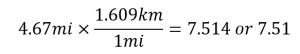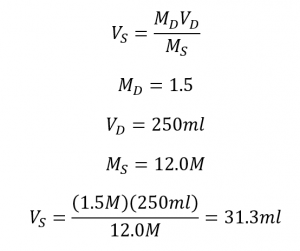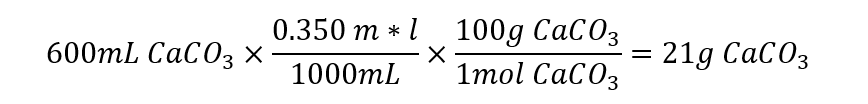# TEAS Science Quiz Answer Explanations

1. What is the total mechanical energy of a system?

Answer: Kinetic energy plus potential energy
Explanation: In any system, the total mechanical energy is the sum of the potential energy and the kinetic energy. Either value could be zero, but it still must be included in the total. The total potential energy and the total kinetic energy only give the total potential or kinetic energy, respectively. Kinetic energy minus potential energy gives the difference in the kinetic and potential energy.

2. In which organelle do eukaryotes carry out aerobic respiration?

Explanation: The mitochondrion is often called the powerhouse of the cell and is one of the most important structures for maintaining regular cell function. It is where aerobic cellular respiration occurs and where most of the cell’s ATP is generated. The number of mitochondria in a cell varies greatly from organism to organism and from cell to cell. Cells that require more energy, like muscle cells, have more mitochondria.

3. In testing how quickly a rat dies by the amount of poison it eats, which of the following is the independent variable and which is the dependent variable?

Answer: Whether the rat eats the poison is the independent variable; how quickly the rat dies is the dependent variable.
Explanation: The independent variable is the variable manipulated and the dependent variable is the result of the changes in the independent variable. “The amount of poison is the independent variable; how quickly the rat dies is the dependent variable.” is correct because the amount of poison is the variable that is changed, and the speed of rat death is the result of the changes in the amount of poison administered. “How quickly the rat dies is the independent variable; the amount of poison is the dependent variable.” is incorrect because that answer states the opposite. “Whether the rat eats the poison is the independent variable; how quickly the rat dies is the dependent variable.” is false because the scientist isn’t attempting to determine whether the rat will die if it eats poison; the scientist is testing how quickly the rat will die depending on how much poison it eats. “The cage the rat is kept in is the independent variable; the amount of poison is the dependent variable.” is incorrect because the cage isn’t manipulated in any way and has nothing to do with the hypothesis.

4. 4.67 miles is equivalent to how many kilometers to three significant digits?

Explanation: The answer choices for this question are tricky. Converting to kilometers from miles will yield the choice 7.514 when using the conversion 1 mile = 1.609 km. However, because the value in miles is written to three significant figures, the answer choice should also yield a value in three significant figures, making 7.51 km the correct answer. 2.90 km and 2.902 km could seem correct if someone flipped the conversion upside-down—that is, if they divided by 1.609 instead of multiplied by it.5. How many mL (to the appropriate number of significant figures) of a 12.0 M stock solution of HCl should be added to water to create 250 mL of a 1.50 M solution of HCl?

Explanation: Preparing a solution from a stock is simply a process of dilution by adding water to a certain amount of the stock. The amount of stock to use can be calculated using a formula and algebra:Because the given values are written to three significant figures, the answer should also be written in three significant figures, making 31.3 mL the correct answer. The other answer choices are either incorrect values or reported to an incorrect number of significant figures.

6. Which is the simplest nerve pathway that bypasses the brain?

Explanation: The reflex arc is the simplest nerve pathway. The stimulus bypasses the brain, going from sensory receptors through an afferent (incoming) neuron to the spinal cord. It synapses with an efferent (outgoing) neuron in the spinal cord and is transmitted directly to muscle. There is no interneuron involved in a reflex arc. The classic example of a reflex arc is the knee jerk response. Tapping on the patellar tendon of the knee stretches the quadriceps muscle of the thigh, resulting in contraction of the muscle and extension of the knee.

7. Which is NOT a function of the pancreas?

Answer: Secretes the hormone insulin in response to growth hormone stimulation
Explanation: The exocrine portion of the pancreas (the majority of it) is an accessory organ to the digestive system (meaning that food never touches it—it is not part of the alimentary canal). It secretes bicarbonate to neutralize stomach acid and enzymes to aid in digestion. It also regulates blood sugar levels through the complementary action of insulin and glucagon that are located in the Islets of Langerhans (endocrine portion). “Secretes the hormone insulin in response to growth hormone stimulation” is incorrect because it is not the growth hormone that stimulates insulin secretion, but rather blood sugar levels.

8. Which muscle system is unlike the others?

Explanation: When muscles contract, they pull bones together. They cannot push apart though, so they work in antagonistic pairs where they are on opposite sides of the bone.

When the bicep contracts, the arm bends and the tricep is relaxed; on the other hand, when the tricep contracts, the arm opens, and the bicep relaxes.

The quadriceps on the thigh straighten the knee; the hamstrings behind the thigh bend the knee.

The trapezius, rhomboid major, and rhomboid minor are muscles on the upper back that pull the shoulders back. The pectoralis major and minor (pecs) are on the chest and allow movement of the shoulder (throwing, lifting, rotating).

The gluteus maximus is the buttocks muscle and extends to the hip. It is the major of the glutes and is responsible for large movements like jumping. The gluteus medius and minimus stabilize the pelvis. The antagonist muscle to the gluteus maximus is the iliopsoas, the flexor muscles. Therefore, Gluteus maximus: Gluteus minimus is correct since the glutes are not antagonistic muscles.

9. Which epithelial tissue comprises the cell layer found in a capillary bed?